## Sunday, October 11, 2009

Implicit Runge-Kutta methods are interesting because you can get high-order accuracy with relatively few stages. A method based on Radau quadrature that is third order accurate and L-stable has only two stages. A comparable multi-step method based on a backward difference that requires saving two steps is only second order accurate.

The Butcher tableau for the method is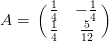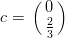The method is applied by calculating several slopes at various points and then adding a weighted sum of them to the initial condition.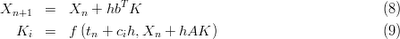The stability of the method can be understood by taking a look at the amplification factor, which for this Runge-Kutta method is given byThe amplification factor in the complex plane for two third-order A-stable Radau-based methods are shown belowThe one on the left (which is the one we're implementing) exhibits stiff decay, otherwise known as L-stability. The amplification factor goes to zero as the max eigenvalue scaled by the time step goes to infinity. We can confirm this with Maxima:

limit((2*z+6)/(z**2-4*z+6), z, minf, plus);

L-stable methods are particularly important for stiff problems. These often occur in chemical kinetics or turbulent flows. Also, Chebyshev pseudospectral methods for PDEs introduce really large eigenvalues which are not very accurately approximated, so damping them is advantageous.

It's easy to apply the method to a linear ODE, such as the second order equation describing the motion of a simple harmonic oscillatorwhich has the analytical solutionand can be written as a first order systemWe can use Maxima's kronecker_product() function to calculate the system operator for both stagesand similarly the operators for the Runge-Kutta integration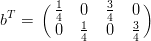Since the system is linear we can actually invert the operator analytically and solve for the update.Which in our case gives the solution at the new time step as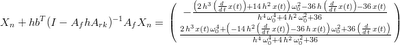Of course the reason this type of time-integration is not very popular is because often you can't analytically invert the system. For nonlinear problems the size of the system that must be iteratively solved is twice as large (for a two-stage method) as the previously mentioned second order backward difference method.

We can dump the update expression to Fortran using Maxima's f90() function, and then compile it to a Python module using F2Py. This makes doing a convergence study in Python pretty easy:

f = 1.0 # frequency
w0 = 2.0*sp.pi*f # angular frequency
np = 1.0 # number of periods
nt = [16, 32, 64, 128, 256] # number of time-steps
h = []
x = []
dxdt = []
t = []
xa = []
err = []
for i in xrange(5):
h.append(np * (1.0/f) / nt[i])
x.append(sp.ones(nt[i], dtype=float))
dxdt.append(sp.zeros(nt[i], dtype=float))
t.append(sp.linspace(0, np-h[i], nt[i]))
xa.append(sho.analytical(x[i], dxdt[i], w0, t[i]))
err.append(sp.sqrt(sum((xa[i] - x[i])**2)/nt[i]))

The numerical solution is compared to the analytical solution for several time-step sizes. The observed order of convergence (2.97) matches the design order (3) pretty closely.Check out Computer Methods for Ordinary Differential Equations and Differential-Algebraic Equations and Solving Ordinary Differential Equations II: Stiff and Differential-Algebraic Problems for some pretty detailed coverage of these and other advanced integration methods. Also see this page with Fortran implementations of several of these types of methods.

1.2.